Next: 2.4 Mesh Generation Up: 2. The Finite Element Previous: 2.2 The Weak Formulation   Contents

# 2.3 Galerkin Discretization

In order to solve the Poisson problem numerically we have to discretize the weak formulation of the Poisson equation (Eq. (2.8)) and restrict the solution space of the numerical solution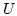to a finite dimensional subspace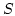of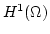. Accordingly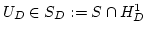approximates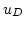on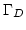. The discretized problem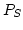can then be written as: Find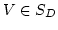such that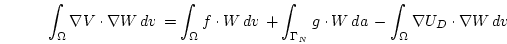(2.9)

with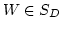.

If we assume that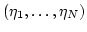is a basis of the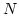-dimensional spaceand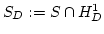a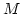-dimensional subspace then we can rewrite Eq. (2.9)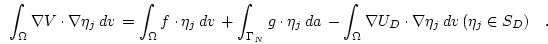(2.10)

If we now make a series expansion of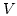and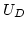in terms of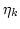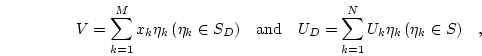(2.11)

then we obtain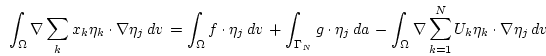(2.12)

which can be rewritten as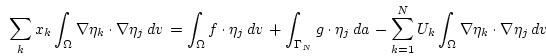(2.13)

and finally simplified to a system of linear equations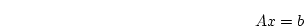(2.14)

where the stiffness matrix'' is given by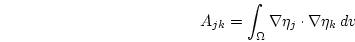(2.15)

and the right hand side'' by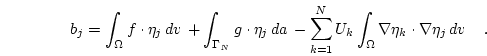(2.16)

The stiffness matrix is sparse, symmetric, and positive definite. Thus, Eq. (2.14) has exactly one solution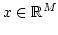, which gives the Galerkin solution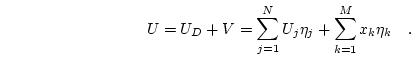(2.17)Next: 2.4 Mesh Generation Up: 2. The Finite Element Previous: 2.2 The Weak Formulation   Contents
Werner Scholz 2003-06-08